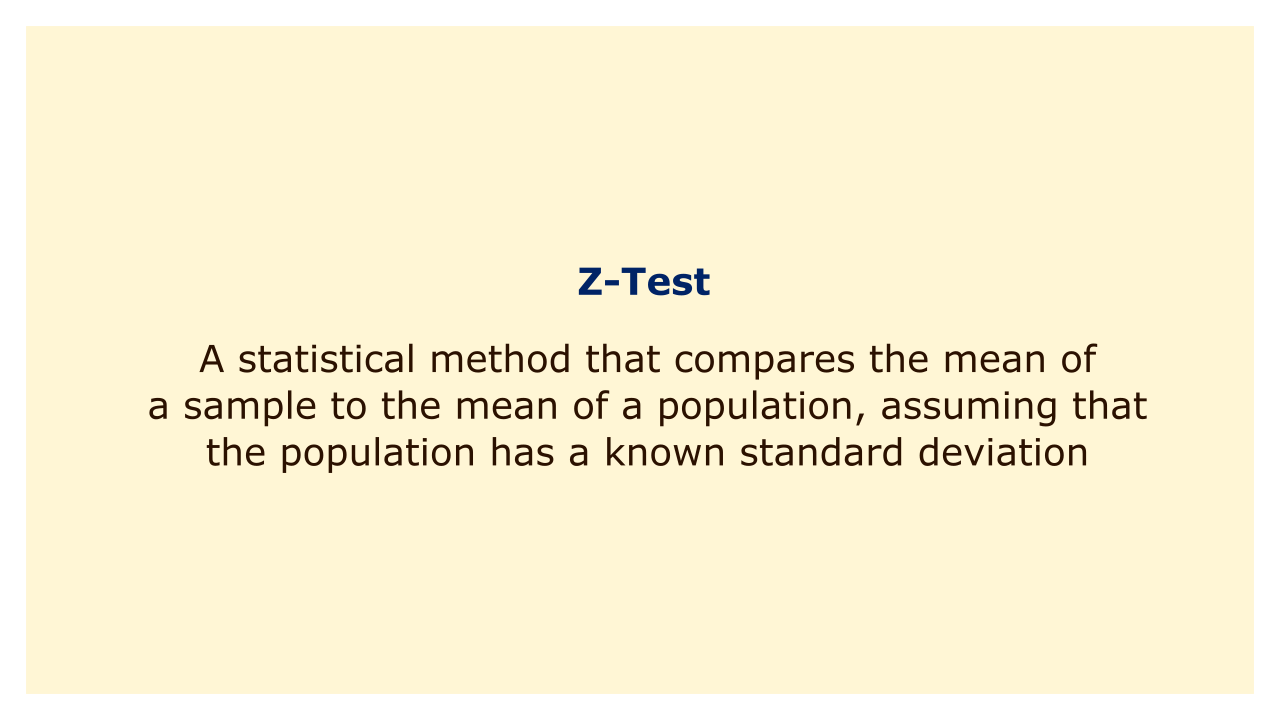# Z-TestImage: Moneybestpal.com

### A z-test is a statistical method that compares the mean of a sample to the mean of a population, assuming that the population has a known standard deviation. A z-test can be used to determine whether or not there is a difference between the sample mean and the population mean.

Calculating a z-score, or the distance between the sample mean and the population mean in standard deviations, is the fundamental concept behind a z-test. Depending on whether the sample mean is higher or lower than the population mean, the z-score might be either positive or negative. The degree of significance and type of test will define the crucial value, which will be used to compare the z-score to (one-tailed or two-tailed).

The level of significance is the probability of rejecting the null hypothesis when it is true, and it is usually denoted by alpha. The alternative hypothesis—which is the null hypothesis's direct opposite—determines the kind of test to be used. In contrast to a two-tailed test, a one-tailed test simply takes into account the greater-than- or less-than-direction of the difference (not equal).

The sample mean, sample size, population mean, and population standard deviation must all be known in order to conduct a z-test. The formula for calculating the z-score is:

Z = (sample mean - population mean) / (population standard deviation / square root of sample size)

After we have the z-score, we may use a calculator or a z-table to compare it to the crucial value. We reject the null hypothesis and come to the conclusion that there is a substantial difference between the sample mean and the population mean if the z-score is more extreme than the critical value. We fail to reject the null hypothesis and come to the conclusion that there is no appreciable difference between the sample mean and the population mean if the z-score is less extreme than the critical value.

Let's examine a z-test usage illustration. Consider the scenario in which we wish to determine whether the average height of pupils in a class differs from 170 cm, the average height of people in their nation. 25 pupils are chosen at random from the class, and we measure their heights. Their standard deviation is 10 cm, and their average height is 168 cm. Assumed to be 10 cm is the standard deviation for the population. Our alternative hypothesis was set to not equal, and our level of significance was set at 0.05.

To perform a z-test, we first calculate the z-score using the formula:

Z = (168 - 170) / (10 / sqrt(25)) = -1

Following that, we use a z-table or a calculator to find the critical value for a two-tailed test with an alpha of 0.05. We discover that it is 1.96.

When we compare our z-score to our critical value, we see that it is -1 1.96, which indicates that we are unable to reject the null hypothesis. We come to the conclusion that the average student height in our class, which is 170 cm, is not significantly different from that.
Tags